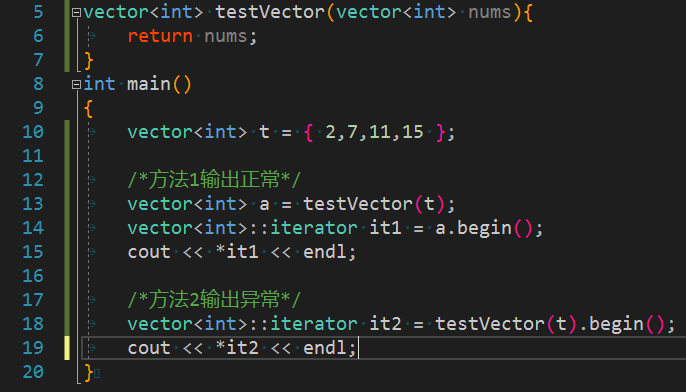C++ vector<int>类型 简单使用迭代器出现异常 不知道是什么原因

vector类型并且原模原样的返回, 最后输出第一个元素的值.``````vector<int> testVector(vector<int> nums){
return nums;
}
int main()
{
vector<int> t = { 2,7,11,15 };

/*方法1输出正常*/
vector<int> a = testVector(t);
vector<int>::iterator it1 = a.begin();
cout << *it1 << endl;

/*方法2输出异常*/
vector<int>::iterator it2 = testVector(t).begin();
cout << *it2 << endl;

}
``````

2个回答

``````// 传入引用举例
vector<int> testVector(vector<int> &nums){
return nums;
}
``````
``````// 传入指针举例
vector<int> testVector(vector<int> *nums){
return *nums;
}
``````

`vector<int>::iterator it2 = testVector(t).begin();`编译器为这一行代码创建了一个`vector<int>` 类型临时对象用于存储`testVector(t)` 的返回结果。`it2`是这个临时对象的迭代器。因表达式求值而创建的临时对象，将在表达式语句的末尾(即分号处)被销毁。当通过`*it2`访问那个临时的`vector<int>` 对象的元素时，此临时对象已经被销毁。有关临时对象的内容，请参考：https://docs.microsoft.com/en-us/cpp/cpp/temporary-objects